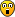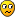Register

## How to make c programs work faster

Learn how to program. Code snippets for creating sprites etc. Submit your own or use those of others.
Senior MemberPosts: 113
Joined: Sun Dec 16, 2012 2:59 pm
Calculators: None

### How to make c programs work faster

Have you ever wondered why your c-programs are slow?
No, you didn't?

Then let us make a speed-test. Run the following code:
code: Show
Code: Select all
`#include "fxlib.h"#include "stdio.h"unsigned int RTC_GetTicks(void);int AddIn_main(int isAppli, unsigned short OptionNum){   double x, y, z;   const char text = {0};   unsigned int key, time;      locate(1, 1);   Bdisp_AllClr_VRAM();      time = RTC_GetTicks();      y = 3;   for(x = 0; x < 0xFF; x++)   {      z = y * x + y;      for(z = 0; z < 0x88; z++);   }      sprintf(&text, "elapsed: %.3f msec", (RTC_GetTicks() - time) * 15.625);   Print(&text);   GetKey(&key);}#pragma section _BR_Sizeunsigned long BR_Size;#pragma section#pragma section _TOPint InitializeSystem(int isAppli, unsigned short OptionNum){return INIT_ADDIN_APPLICATION(isAppli, OptionNum);}#pragma section`

My result: 531.25 msecs.

Now let's do this with floats instead of doubles:
Code: Select all
`   float x, y, z;`

My result: 328.125 msecs - that's a lot faster than with doubles!

Another test, with integers:
code: Show
Code: Select all
`int AddIn_main(int isAppli, unsigned short OptionNum){   int x, y, z, c;   const char text = {0};   unsigned int key, time;      locate(1, 1);   Bdisp_AllClr_VRAM();      time = RTC_GetTicks();      y = 3;   for(c = 0; c < 0x4F; c++)   {      for(x = 0; x < 0xFF; x++)      {         z = y * x + y;         for(z = 0; z < 0x88; z++);      }   }      sprintf(&text, "elapsed: %.3f msec", (RTC_GetTicks() - time) * 15.625);   Print(&text);   GetKey(&key);}`

My result: 765.625 msecs (If you want to compare this with floats and doubles you have to divide it through 0x4F = 79, ~9.691).

Code: Select all
`   long x, y, z, c;`

My result: 765.625 (compare: 9.691)

Let's test short integers:
Code: Select all
`   short x, y, z, c;`

My result: 1109.375 (compare with the others: ~14.043)Now chars:
code: Show
unsigned char x, y, z, c;
const char text = {0};
unsigned int key, time;

locate(1, 1);
Bdisp_AllClr_VRAM();

time = RTC_GetTicks();

y = 3;
for(c = 0; c < 0xF; c++)
{
for(x = 0; x < 0xFF; x++)
{
z = y * x + y;
for(z = 0; z < 0x88; z++);
}
}

sprintf(&text, "elapsed: %.3f msec", (RTC_GetTicks() - time) * 15.625);
Print(&text);
GetKey(&key);
Code: Select all

My result: 250 msecs (compare: 15.625)So here are all results:

double: 531.25
float: 328.125
char: 15.625
short: 14.043
long / int: 9.691

So, why is double slower than float?

Run this code:
code: Show
Code: Select all
`#include "fxlib.h"#include "stdio.h"int AddIn_main(int isAppli, unsigned short OptionNum){   const char text = {0};   unsigned int key;      Bdisp_AllClr_VRAM();      sprintf(&text, "double: %d, float: %d", sizeof(double), sizeof(float));   locate(1, 1);   Print(&text);      sprintf(&text, "long: %d, int: %d", sizeof(long), sizeof(int));   locate(1, 2);   Print(&text);      sprintf(&text, "short: %d, char: %d", sizeof(short), sizeof(char));   locate(1, 3);   Print(&text);   GetKey(&key);}#pragma section _BR_Sizeunsigned long BR_Size;#pragma section#pragma section _TOPint InitializeSystem(int isAppli, unsigned short OptionNum){return INIT_ADDIN_APPLICATION(isAppli, OptionNum);}#pragma section`

It outputs the size of the types.

double: 8
float: 4
long / int: 4
short: 2
char: 1

Now let's think about the results of the tests:
double: 531.25 msecs, 8 bytes
float: 328.125 msecs, 4 bytes
char: 15.625 msecs, 1 byte
short: 14.043 msecs, 2 bytes
long / int: 9.691, 4 bytes

Short info: the SH3 / SH4 is a 32 bit (4 byte) processor.

That means that the processor can load a 4-byte-variable at once (float, long / int). Of course the floating point types are slower, so the integer is the fastest variable type.

Senior MemberPosts: 113
Joined: Sun Dec 16, 2012 2:59 pm
Calculators: None

### How to make c programs work faster - bit operations

I've got another trick:
code: Show
Code: Select all
`#include "fxlib.h"#include "stdio.h"unsigned int RTC_GetTicks();int AddIn_main(int isAppli, unsigned short OptionNum){   const char text = {0};   unsigned int key, time;   int x, y, z;      Bdisp_AllClr_VRAM();   locate(1, 1);      time = RTC_GetTicks();      z = y = 2;      for(x = 0; x < 0xFF; x++)   {      for(y = 0; y < 0xFFF; y++)      {         z = y * 64;      }   }      sprintf(&text, "elapsed: %.3f msecs", (RTC_GetTicks() - time) * 15.625);   Print(&text);      GetKey(&key);}#pragma section _BR_Sizeunsigned long BR_Size;#pragma section#pragma section _TOPint InitializeSystem(int isAppli, unsigned short OptionNum){return INIT_ADDIN_APPLICATION(isAppli, OptionNum);}#pragma section`

My result: 296.875 msecs

Now we use shift left to multiple:
Code: Select all
`         z = y << 6;`

My result: 281.25 msecs

Conclusion: Shift operations are a bit () faster than "normal" operators.

Now another question: What's faster? The preprocessor or a function?
code: Show
Code: Select all
`#include "fxlib.h"#include "stdio.h"unsigned int RTC_GetTicks();int dosomething(int a){   return (a + 12345 - 9876) / 123;}int AddIn_main(int isAppli, unsigned short OptionNum){   const char text = {0};   unsigned int key, time;   int x, y, z;      Bdisp_AllClr_VRAM();   locate(1, 1);      time = RTC_GetTicks();      z = y = 2;      for(x = 0; x < 0xFF; x++)   {      for(y = 0; y < 0xFF; y++)      {         z = dosomething(y);      }   }      sprintf(&text, "elapsed: %.3f msecs", (RTC_GetTicks() - time) * 15.625);   Print(&text);      GetKey(&key);}#pragma section _BR_Sizeunsigned long BR_Size;#pragma section#pragma section _TOPint InitializeSystem(int isAppli, unsigned short OptionNum){return INIT_ADDIN_APPLICATION(isAppli, OptionNum);}#pragma section`

My result: 453.125 msecs

Now we write
Code: Select all
`#define dosomething(a) (a + 12345 - 9876) / 123`

Code: Select all
`int dosomething(int a){   return (a + 12345 - 9876) / 123;}`

and
Code: Select all
`    for(x = 0; x < 0xFFF; x++)`

Code: Select all
`    for(x = 0; x < 0xFF; x++)`

My result: 296.875 msecs (compare it: 296.875 / 16 = ~ 18.555)

preprocessor: 18.55 msecs
function: 453.125 msecs

That means that a function is much slower than the preprocessor directive (the processor does'nt have to allocate new memory etc.).

Senior MemberPosts: 605
Joined: Sat Sep 15, 2012 6:59 am
Location: Krautland ****
Calculators: Casio fx-7400GII, Casio fx-7400GII (SH4), Casio fx-9750GII, Casio fx-9750GII (SH4), Casio fx-9860G, Casio fx-9860G SD, Casio fx-9860G Slim, Casio fx-9860GII SD, Casio fx-9860GII SD Power Graphic 2, Casio Classpad 330 plus, Casio fx-CG20, Casio fx-CG50, Casio Classpad fx-CP400

### Re: How to make c programs work faster

As for square roots:
Code: Select all
`unsigned int i_sqrt( unsigned int op ){    unsigned int res = 0;    unsigned int one = 1 << 30;    // "one" starts at the highest power of four <= than the argument.    while (one > op) one >>= 2;    while (one != 0){        if (op >= res + one){            op -= res + one;            res += one << 1;        }        res >>= 1;        one >>= 2;    }    /* Do arithmetic rounding to nearest integer */    if (op > res) res++;    return res;}`
This is ten times faster than the float or double variant.
Found it here
I'll be back!